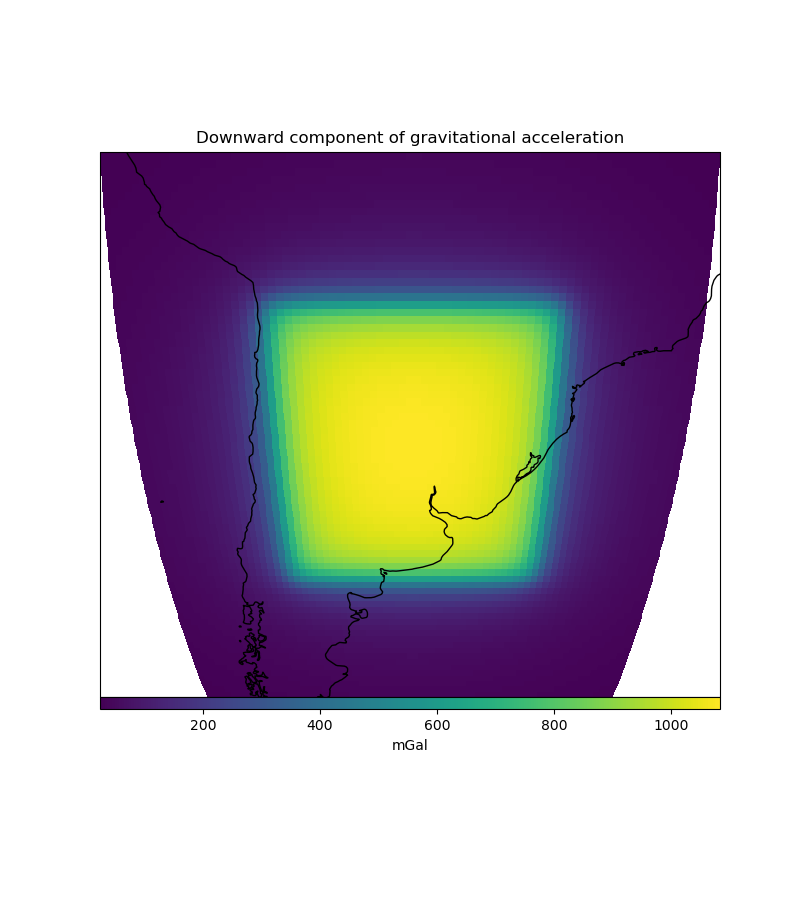# Tesseroids (spherical prisms)¶

Computing the gravitational fields generated by regional or global scale structures require to take into account the curvature of the Earth. One common approach is to use spherical prisms also known as tesseroids. We will compute the downward component of the gravitational acceleration generated by a single tesseroid on a computation grid through the `harmonica.tesseroid_gravity` function.Out:

```[[26.25264292 26.6161005  26.98494351 ... 26.98494351 26.6161005
26.25264292]
[26.82151989 27.2134279  27.61202586 ... 27.61202586 27.2134279
26.82151989]
[27.41031127 27.8332173  28.26436726 ... 28.26436726 27.8332173
27.41031127]
...
[24.3828477  24.83864142 25.30833989 ... 25.30833989 24.83864142
24.3828477 ]
[23.90092716 24.33039969 24.77197999 ... 24.77197999 24.33039969
23.90092716]
[23.43483023 23.83981484 24.25533515 ... 24.25533515 23.83981484
23.43483023]]
/usr/share/miniconda3/envs/testing/lib/python3.8/site-packages/cartopy/mpl/geoaxes.py:1797: MatplotlibDeprecationWarning: shading='flat' when X and Y have the same dimensions as C is deprecated since 3.3.  Either specify the corners of the quadrilaterals with X and Y, or pass shading='auto', 'nearest' or 'gouraud', or set rcParams['pcolor.shading'].  This will become an error two minor releases later.
result = matplotlib.axes.Axes.pcolormesh(self, *args, **kwargs)
```

```import matplotlib.pyplot as plt
import cartopy.crs as ccrs
import verde as vd
import boule as bl
import harmonica as hm

# Use the WGS84 ellipsoid to obtain the mean Earth radius which we'll use to
# reference the tesseroid
ellipsoid = bl.WGS84

# Define tesseroid with top surface at the mean Earth radius, thickness of 10km
# (bottom = top - thickness) and density of 2670kg/m^3
density = 2670

# Define computation points on a regular grid at 100km above the mean Earth
coordinates = vd.grid_coordinates(
region=[-80, -40, -50, -10],
shape=(80, 80),
)

# Compute the radial component of the acceleration
gravity = hm.tesseroid_gravity(coordinates, tesseroid, density, field="g_z")
print(gravity)

# Plot the gravitational field
fig = plt.figure(figsize=(8, 9))
ax = plt.axes(projection=ccrs.Orthographic(central_longitude=-60))
img = ax.pcolormesh(*coordinates[:2], gravity, transform=ccrs.PlateCarree())
plt.colorbar(img, ax=ax, pad=0, aspect=50, orientation="horizontal", label="mGal")
ax.coastlines()
ax.set_title("Downward component of gravitational acceleration")
plt.show()
```

Total running time of the script: ( 0 minutes 5.998 seconds)

Gallery generated by Sphinx-Gallery